# General position subsets

 Importance: Medium ✭✭
 Author(s): Gowers, Timothy
 Subject: Geometry
 Keywords: general position subset, no-three-in-line problem
 Posted by: David Wood on: March 24th, 2014
Question   What is the least integersuch that every set of at leastpoints in the plane containscollinear points or a subset ofpoints in general position (no three collinear)?

The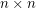grid contains no set of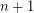collinear points and no subset ofpoints in general position, implying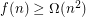.

To see that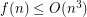, consider a set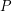of points that contain nocollinear points, and contain no subset ofpoints in general position. Letbe a maximal subset ofin general position. Every point in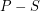is on one of thelines determined by. Each such line contains at mostpoints in. Thus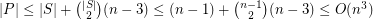.

Payne and Wood [PW] improved this upper bound to. The proof is based on the Szemerédi-Trotter Theorem and Spencer's Lemma about independent sets in hypergraphs.

It is reasonable to think that the grid is the extremal example, and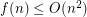. This would be an elegant generalisation of a result by Erdős [R] who proved that thegrid contains a subset of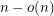points in general position (the no-three-in-line problem).

## Bibliography

*[G] Timothy Gowers, A geometric Ramsey problem.

[PW] Michael Payne, David R. Wood. On the general position subset selection problem, SIAM J. Discrete Math. 27.4:1727-1733, 2013.

[R] K. F. Roth, On a problem of Heilbronn, J. London Mathematical Society 26.3:198–204, 1951.

* indicates original appearance(s) of problem.